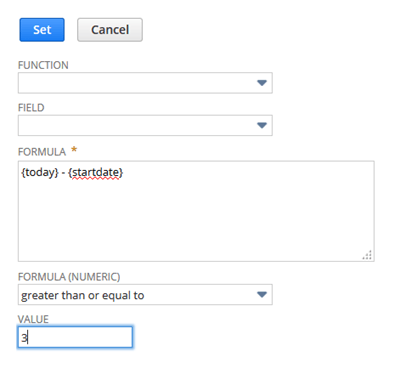# Using a Formula in Search Criteria

#### To use a formula in search criteria:

1. On the Criteria subtab of an advanced or saved search page, in the Filter column, select the required Formula type.

The following options are available (they are listed in alphabetical order, intermingled with field names in the Filter column dropdown list):

• Formula (Date)

• Formula (Numeric)

• Formula (Text)

The best choice for a formula type depends upon the calculation the formula will perform, because validation varies according to the formula type. For example, to do character manipulation using regular expressions, you should select Formula (Text). To do numeric calculations, Formula (Numeric) is likely a better choice.

To include both positive and negative values in search results when using Quantity, set Formula (Numeric) in the Filter field and `{Quantity}` in the Formula field. If you use Quantity in the Filter field, you will receive only positive values in search results. All negative results are automatically filtered out.

Important:

To avoid cross-scripting (XSS) vulnerabilities, search column results with formulas that contain <script> tags do not display script output. Do not use <script> tags in your search formulas. To avoid any HTML output from being rendered, you can check the Disable HTML in Search Formula (Text) box at Setup > Company > General Preferences. For more information, see Restricting HTML for Search Formulas.

2. In the Formula popup window, define your formula, and click Done.

Note:

For details about supported SQL functions, refer to SQL Expressions . For tables of NetSuite field IDs, you can also refer to SuiteScript Supported Records.

• Options vary depending on the type of formula selected. For example, date formulas can be filtered based on whether the date occurs before or after a specified date, whereas numeric formulas can be filtered based on whether the resultant value is greater than or equal to the specified criteria.

• Select from the Function dropdown list to include a SQL function in the formula expression.

• Select a field name from the Filter dropdown list to include its ID in the formula expression.

• There is a 1000 character limit per formula expression. You may be able to preview a search with a formula containing more characters, but when you run the search invalid expression errors occur.

• Remember that search records are dynamically filtered based on the calculated value of the formula at the time the search is performed.

3. Click Submit to view your search results.

For example, select Formula (Numeric) and enter the formula {today} - {startdate}, then set the criteria as greater than or equal to 3, as shown in the following figure.